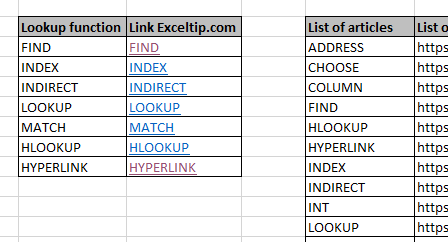# How to link the first match in ExcelScenario :

When working with long data with links. We need to get the link as a hyperlink with the matched value. What is hyperlink? And How to add a matched link in the cell? A hyperlink is simply a link, is a reference to data that the user can follow by clicking or tapping. Data can be any document or indicated part of a document. The text that is linked from is called anchor text. Below here is the formula and example explanation to lookup match and hyperlink the corresponding value.

How to solve the problem?

For this, we will be using the HYPERLINK function and VLOOKUP function. From the given table we need to find the match link and hyperlink with anchor text. So anchor text will be the lookup value and VLOOKUP returns the address to the matched value. And the HYPERLINK function attaches the address to the anchor text.

Generic formula:

 =HYPERLINK ( VLOOKUP ( anchor_text , table_array , col , 0 ), anchor_text)

anchor_text : value to lookup and text where link is attached

table_array : lookup table array

col : column index number, list of links

0 : lookup exact match

Example :

All of these might be confusing to understand. So Let's understand this formula using it in an example stated below. Here we have taken some function article list with links in a table and we need to get the lookup value as anchor text and address as hyperlink. Below is the formula with explanation to use. Here Table is the named range used for the table array (E4:F19).

Use the formula:

 =HYPERLINK( VLOOKUP( B4 , Table, 2, 0), B4)

Explanation:

1. VLOOKUP function lookup "FIND" in the first column (E4:E19) of the Table array (E4:F19).
2. Then the function looks up the corresponding result link in 2nd Columnand returns the link.
3. 0 argument, to look up the exact match.
4. Now the HYPERLINK function adds the link to the anchor text, which is the same as the lookup value argument.As you can see we obtained anchor text "FIND" with the address link of the Find function in Excel. Now copy the formula to other cells using the shortcut key Ctrl + D or dragging down from the right bottom of the C4 cell.In the above snapshot,  all the lookup values got the links attached to it. Now Clicking or tapping any of the obtained values will open the article linked in the default browser selected. For example, If HYPERLINK is clicked. It will open the article as shown below.Here is the Exceltip link, How to use the HYPERLINK function in Excel. You can view the linked link by just taking your mouse pointer to the text value. It will become hand pointer and a white box appears as shown below.Customize this formula with other links and anchor text and have your own bookmarks in Excel list. The Lookup table can be of any length, this formula works fine. You can also use the combination of the INDEX and MATCH function here.

Here are all the observational notes regarding using the formula.

Notes:

1. This formula works with text and numbers both.
2. The link will not redirect, if it is not valid. Excel throws an error "Cannot open the specified file", if you tap on any invalid link.
3. Lookup value must be in the column of the table or else the VLOOKUP function return error and that will be linked to the anchor text.

Hope you understood How to link the first match in Excel. Explore more articles on Excel lookup and match formulas here. If you liked our blogs, share it with your friends on Facebook. And also you can follow us on Twitter and Facebook. We would love to hear from you, do let us know how we can improve, complement or innovate our work and make it better for you. Write to us at info@exceltip.com.

Related Articles :

Use INDEX and MATCH to Lookup Value : combination of INDEX & MATCH function formula to look up value in table as required in Excel.

SUM range with INDEX in Excel : Use INDEX function to find the SUM of the values as required.

How to use the INDEX function in Excel : Find the INDEX of an array using the INDEX function explained with example.

How to use the MATCH function in Excel : Find the MATCH in the array using the INDEX value inside MATCH function explained with example.

How to use LOOKUP function in Excel : Find the lookup value in the array using the LOOKUP function explained with example.

Popular Articles:

How to use the IF Function in Excel : The IF statement in Excel checks the condition and returns a specific value if the condition is TRUE or returns another specific value if FALSE.

How to use the VLOOKUP Function in Excel : This is one of the most used and popular functions of excel that is used to lookup value from different ranges and sheets.

How to use the COUNTIF Function in Excel : Count values with conditions using this amazing function. You don't need to filter your data to count specific values. Countif function is essential to prepare your dashboard.

How to use the SUMIF Function in Excel : This is another dashboard essential function. This helps you sum up values on specific conditions.

Terms and Conditions of use

The applications/code on this site are distributed as is and without warranties or liability. In no event shall the owner of the copyrights, or the authors of the applications/code be liable for any loss of profit, any problems or any damage resulting from the use or evaluation of the applications/code.